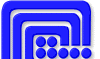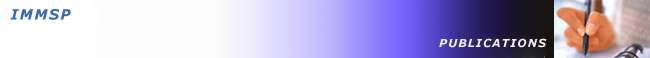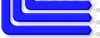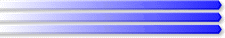ukrainian version

Mathematical Machines and Systems. 2013 #1

ABSTRACTS

### COMPUTER SYSTEMS

UDC 004.3

Carbon nanoelectronic element base of informatics. P. 1 / Voitovych I.D.,
Korsunsky V.M., Lavryk F.T. // Mathematical Machines and Systems. - 2013. - N 1. - P. 3 - 13.

This paper introduces readers with up to date element base which can replace silicon element base of informatics. The properties of fullerenes, carbon nanotubes (CNT), graphene films were briefly described as well as transistors, logic circuits, resistors, interconnections, memory cells, ultra-sensitive sensors and display devices were realized on their base. The advantages and prospects of implementation of such elements in the nearest decades were pointed out. Figs.: 9. Refs.: 38 titles.

UDC 519.2: 530.1: 600.1

The sixth D. Gilbert's problem: role and value of physical hypotheses / Gorban I.I. // Mathematical Machines and Systems. - 2013. - N 1. - P. 14 - 20.

In present, a lot of disciplines, per se physical ones (the probability theory and the rational mechanics, in particular) are presented as the abstract mathematical theories. Therefore the sixth D. Gilbert's problem connected with axiomatization of physical disciplines has not solved so far. For physical disciplines, for which axiomatic mathematic theories are created the solution of the problem can be provided by the additional physical hypotheses (the adequacy axioms) that fix the presence and the particularities of the base of physical phenomena. Demands for the physical hypotheses are formulated. Fig.: 1. Refs.: 11 titles.

UDC 511.1, 512.542, 530.145

Differential operator in the system of abstract register      transformations /
Belyaev A.K., Klymenko V.P. // Mathematical Machines and Systems. - 2013. - N 1. - P. 21 - 25.

We consider a special differential operator for constructing and representation of arithmetic functions in the system of transformations of an abstract register. The possibility of structural buildings and performing of precise calculations are determinated. Refs.: 6 titles.

UDC 004.519.712 +004.92

Problem solution of the intersection of  convex polyhedrons / Tereschenko V.M. // Mathematical Machines and Systems. - 2013. - N 1. - P. 26 - 33.

In this paper we consider the modified version of GJK algorithm for finding a common point of two convex polyhedrons. Knowing this point and using the duality theorem, we can find the intersection of two polytopes. Considering the polytopes in pairs, we can find an intersection of  polytopes. A parallel software implementation of problem solution which effectively utilizes resources of modern multiprocessor systems was developed. Figs.: 2. Refs.: 13 titles.

UDC 519.765:004.89

The analysis of semantic images in the text objects arrays by quantum calculations / Pavlyshenko B.M. // Mathematical Machines and Systems. - 2013. - N 1. - P. 34 - 43.

The analysis of semantic patterns in the text objects arrays using Grover algorithms elements has been performed. It is shown that implementation of quantum algorithms for some classes of problems gives the ability to decrease required memory exponentially and decrease the time of algorithm performance polynomial in comparison with the classical algorithms due to quantum parallelism. Refs.: 10 titles.

UDC 004.89

The effectiveness of ontological models usage for software systems design /
Burov Ye.V. // Mathematical Machines and Systems. - 2013. - N 1. - P. 44 - 55.

The results of effectiveness analysis of ontology models usage for software systems design are presented. The effectiveness is considered as software quality increase due to ontological models usage on all parts of its life-cycle. Metrics for measuring software quality increase for different sources and stages of life-cycle of software product are suggested. Tabl.: 1. Figs.: 2. Refs.: 15 titles.

UDC 004.93

Extraction method of training samples from the original samples of large volume for patterns diagnosis and recognition / Subbotin S.О. // Mathematical Machines and Systems. - 2013. - N 1. - P. 56 - 62.

The task of sample formation automaticity for diagnostic and recognizing model building on precedents is solved. Extraction method of training samples is offered. It maintains saving the important topological properties of the original sample in a formed sub-sample, and does not require download of the original sample to the computer memory, and the numerous passages of the original sample. This reduces the size of the sample and reduces the resource requirements of a computer. Tabl.: 1. Refs.: 15 titles.

UDC 681.3

Methods of parallel solution of Ax=b systems in a single technological flow of mathematical physics problems solution / Ledyankin Yu.Ya. // Mathematical Machines and Systems. - 2013. - N 1. - P. 63 - 74.

The ways of solving  Ax=b systems for special parallel type processor on the base of processing elements (PE) with a scalar multiplier (SM) within each PE are suggested. Matrix factorization of  p*p size is suggested to perform by the methods allowing to reduce the number of divisions operations on the direct route of exceptions in n-times. On the return route of calculation of indeterminate it is proposed to exclude a division, performing in PE on SM only the operations of multiplication and totalling. Refs.: 8 titles.

INFORMATION AND TELECOMMUNICATION TECHNOLOGY

UDC 681.5:658.149.3(477)

Experience of creating of national integrated system of technology transfer in Ukraine / Lysenko V.S., Yegorov S.O., Grytsay A.Ya., Rudnytsky Ye.A. // Mathematical Machines and Systems. - 2013. - N 1. - P. 75 - 82.

The objects of technologies, technologies and their components as the objects of commercialization process (technology transfer) are regarded in the paper. Information components of these objects are defined by principle operative factors of technologies promoting to the market. The authors propose to consider a special informational component of object transfer technology, which combines scientific and technical capabilities, business interests and market demands, - technology profile. Integrating of National Bank of technology with transfer network technology allows to fill with content the object transfer technology (to determine the components and technology objects), ranging from the moment of creative idea appearance to implementation it into the ultimate consumer. Tabl.: 1. Figs.: 2. Refs.: 25 titles.

UDC 621.391

Information technology of OFDM-FHSS system construction based on optimal time-frequency signal-code structures / Zaitsev S.V., Yarylovets A.V., Nazaruk V.D. / Mathematical Machines and Systems. - 2013. - N 1. - P. 83 - 93.

Information technology of OFDM-FHSS system construction based on optimal time-frequency sequences and Reed-Solomon codes was designed in the paper. The usage of developed information technology in practice will improve noise immunity and reduce the complexity of radio communications used OFDM-FHSS technology. Tabl.: 2. Figs.: 3. Regs.: 6 titles.

UDC 004.416.3; 004.855.5

Usage of mathematical logic for training regime establishing in automation systems of knowledge transfer / Fedoruk P.I., Pikulyak M.V. // Mathematical Machines and Systems. - 2013. - N 1. - P. 94 - 101.

Usage of apparatus of mathematical logic in the programming problems was analyzed and the effectiveness of three-digit mathematical logic for scenario examples processing with the purpose of building adaptive learning trajectory in automated systems of knowledge transfer was investigated. Tabl.: 1. Figs.: 3. Refs.: 12 titles.

UDC 004.896: 004.942

Formation of a control strategy of process module according to current monitoring and express-diagnosis data / Yarmilko A.V. // Mathematical Machines and Systems. - 2013. - N 1. - P. 102 - 110.

The article introduces the concept and methodology of formation of the cost-effective control strategy of process module on the base of combining of adaptability and optimality principles. The theoretical and practical aspects of its implementation are considered. The results of simulation modeling process of determining of the current strategies under the re-creation of manufacturing situation are represented. Tabl.: 1. Figs.: 6. Refs.: 12 titles.

### SIMULATION AND MANAGEMENT

UDC 681.5.015

Formalization of the process of geometric models creation / Lytvynov V.V.,
Khomenko I.V. // Mathematical Machines and Systems. - 2013. - N 1. - P. 111 - 117.

The paper deals with the formalization of the process of geometric models creation and graphic representation of data and knowledge in the systems for automated learning. We presented examples of model building of bodies of simple shapes using binary operations; also we examined their properties and geometric interpretation. Morgan laws were presented and proved, distributive operations applied to operations with solids. Figs.: 6. Refs.: 6 titles.

UDC 004.942

Modeling and visualization by Processing methods of Computer Networks /
Pasichnyk V.V., Ivanuschak N.M. // Mathematical Machines and Systems. - 2013. - N1. - P. 118 - 126.

The paper presents the investigation of computer internet-networks, based on the concept of statistical physics of complex networks, theoretical studies and simulations using mathematics as a tool and analysis language; the technique was considered and modeling of the growth and structuring of local networks was performed. The results of this growth are coordinated with the empirical data. Tabl.: 2. Figs.: 5. Refs.: 13 titles.

UDC 622.24.05-004.4

Modelling of ultrasonic response from the major pipeline wall with insulating coating by the transfer matrix method / Mandra A.A., Lyutak I.Z., Lyutak Z.P. // Mathematical Machines and Systems. - 2013. - N 1. - P. 127 - 136.

The transfer matrix method for modeling of acoustic vibration response of ultrasonic range from the pipeline wall covered by an insulating coating is proposed. The structure of transfer matrices elements is identified. The effect of non-ideal conditions of mechanical excitation of ultrasonic wave by transducer is taken into account. Comparison of modeling results of the ultrasonic vibrations propagation in three environments is carried out. The results of modelling are presented as diagrams. Таbl.:1. Figs.: 2. Refs.: 12 titles.

UDC 004.942

Model of optimum order size of goods on credit taking into account receiving inspection errors / Yegorova O.V., Snytyuk V.Ye. // Mathematical Machines and Systems. - 2013. - N 1. - P. 137 - 149.

In this paper inventory management problem with permissible delay in payments is discussed. Inventory management models based on incoming inspection errors in which the deficit is not covered or covered with subsequent deliveries wholly or partially are suggested. Numerical examples of modeling results are given. Tabl.: 5. Figs.: 3. Refs.: 14 titles.

UDC 519.246.8

The basic concepts of multiple regression analysis / Radchenko S.G., Lapach S.M. // Mathematical Machines and Systems. - 2013. - N 1. - P. 150 - 156.

The typical conditions for statistic regressive models formation were described. The methods for models with the possibly optimal statistical properties were developed. The specific system solutions for the key problems of regression analysis and directions for future research were described. Refs.: 11 titles.

QUALITY, RELIABILITY AND CERTIFICATION OF COMPUTER TECHNIQUE AND SOFTWARE

UDC 681.18.656.25

Radiomikroprocessor system of automatic crossing-alarm at railway transport / Fedukhin O.V. // Mathematical Machines and Systems. - 2013. - N 1. - P. 157 - 162.

This article is devoted to the problem of traffic safety at railway crossings. The building concept of a microprocessor dependable system of automatic crossing-alarm with data transmission over the radio channel was investigated. Figs.: 3. Refs.: 5 titles.

UDC 621.3.019.3

Modeling of reliability of nonrestorable quasibridge structure / Pasko V.P. // Mathematical Machines and Systems. - 2013. - N 1. - P. 163 - 171.

The questions of statistical modelling of quasibridge structure reliability are considered on the basis of generators of the random numbers having -distribution. The algorithm of reliability modelling and examples of systems reliability modeling for the various initial data on reliability parameters of components are offered. Tabl.: 2. Figs.: 6. Refs.: 3 titles.